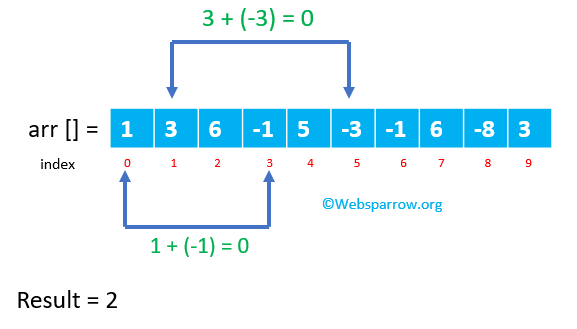# Java- Count number of pairs in the Array whose sum is zero

This Java example will help to solve a given scenario.

Scenario: Write down a Java program that calculates the number of pairs in the array. Pair is when two numbers are added together, and the result is zero. Consider only the first occurrence of each pair.Solution:

1. Remove all duplicate elements from the array.
2. Iterate the elements and check whether it’s positive/negative elements available in the array.
3. If available, remove that element from the array and increment the counter value by 1.
PairCount.java
``````package org.websparrow;

import java.util.Arrays;
import java.util.List;
import java.util.Set;
import java.util.concurrent.CopyOnWriteArraySet;

public class PairCount {

public static void main(String[] args) {

// Test case 1
Integer[] testCase1 = new Integer[]{1, 3, 6, -1, 5, -3, -1, 6, -8, 3};
int testCase1Result1 = countPair(Arrays.asList(testCase1));
System.out.println("Total numbers of pair for test case 1: " + testCase1Result1);

// Test case 2
Integer[] testCase2 = new Integer[]{1, 1, 1, 1, 1, 1, 1, 1};
int testCase1Result2 = countPair(Arrays.asList(testCase2));
System.out.println("Total numbers of pair for test case 2: " + testCase1Result2);

// Test case 3
Integer[] testCase3 = new Integer[]{1, 2, 3, 4, 1, -1, -1, -2, 2, -2, 3, 4, -4, 11, -5, 9, -5};
int testCase1Result3 = countPair(Arrays.asList(testCase3));
System.out.println("Total numbers of pair for test case 3: " + testCase1Result3);
}

public static int countPair(List<Integer> numbers) {
int count = 0;
Set<Integer> uniqueNumbers = new CopyOnWriteArraySet<>(numbers);
System.out.println("Unique numbers: " + uniqueNumbers);
for (int num : uniqueNumbers) {
if (uniqueNumbers.contains(-num)) {
uniqueNumbers.remove(num);
count++;
}
}
return count;
}
}``````

Result:

``````Unique numbers: [1, 3, 6, -1, 5, -3, -8]
Total numbers of pair for test case 1: 2

Unique numbers: 
Total numbers of pair for test case 2: 0

Unique numbers: [1, 2, 3, 4, -1, -2, -4, 11, -5, 9]
Total numbers of pair for test case 3: 3``````

### Reference

#### Similar Posts